8.10

## Lecture 10: Proper Tail Calls on x64

This is the counterpart to Lecture 9, using the x64 calling convention instead of the stack-only calling convention. Most of the material is the same, so we focus primarily on the stack-layout diagrams and on the details of the tail-calling code itself.

### 1Examining the stack

Let’s consider what the stack looks like while evaluating fact_v1. (As before, in this diagram, colors indicate which stack frame uses a particular value on the stack, while the brackets indicate which stack frame created a particular value on the stack.)

 In top-level In fact_v1(4) In fact_v1(3) In fact_v1(2) In fact_v1(1) At fact_v1(4) At fact_v1(3) At fact_v1(2) At fact_v1(1) About to return RDI = ?? RDI = 4 RDI = 3 RDI = 2 RDI = 1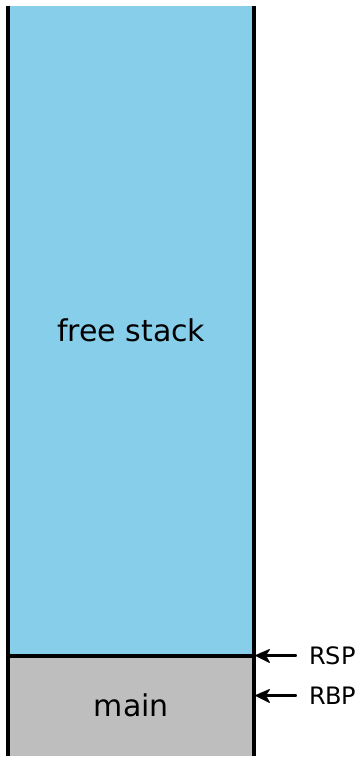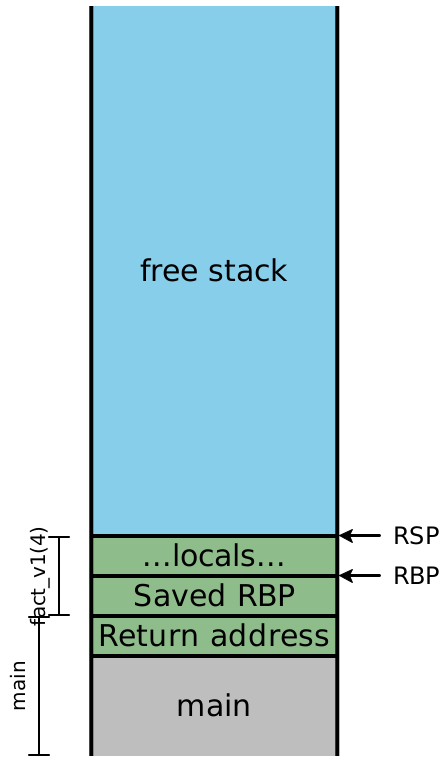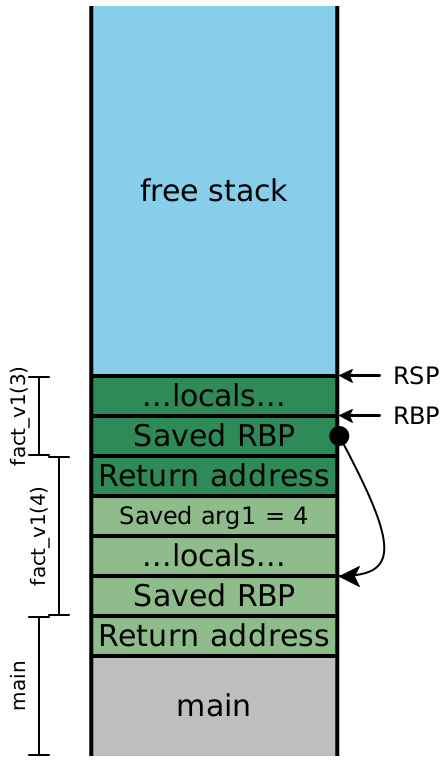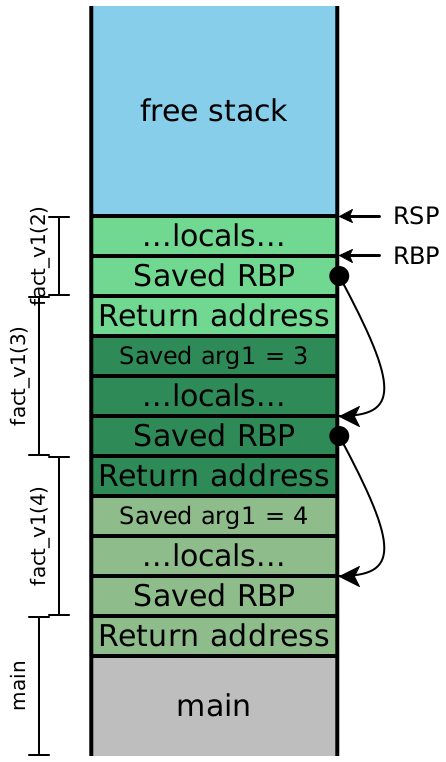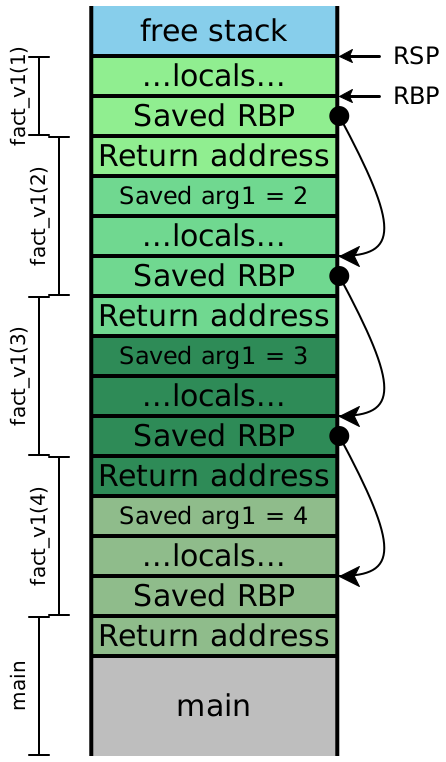Note: The example code above uses functions with only one argument, so no function arguments ever got pushed onto the stack for a given function call. However, the first argument to our functions is passed in RDI, which is a caller-saved register that is going to be needed after the call returns: it needs to be saved (onto the stack) before each function call so that it can be restored and used afterward. In general, with larger-arity functions, our stack picture will contain arguments 7 and up, spliced in between the “Saved arg1” slot of one stack frame and the “Return address” slot of the next stack frame. Additionally, our diagram only tracks the value of RDI; if the functions needed more arguments, we’d need to keep track of the remaining argument registers (RSI, RDX, RCX, R8 and R9), and we’d need to save those values onto the stack as well. The specific order of the saved arguments on the stack doesn’t matter; we just need to pick a location for each saved value and be consistent about it.

Now let’s examine the stacks for fact_v2, assuming we compile our code exactly as we’ve always been. We’ll include the local variables, this time:

 In top-level In fact_tail(4, 1) In fact_tail(3, 4) In fact_tail(2, 12) In fact_tail(1, 24) At fact_tail(4, 1) At fact_tail(3, 4) At fact_tail(2, 12) At fact_tail(1, 24) About to return RDI = ??, RSI = ?? RDI = 4, RSI = 1 RDI = 3, RSI = 4 RDI = 2, RSI = 12 RDI = 1, RSI = 24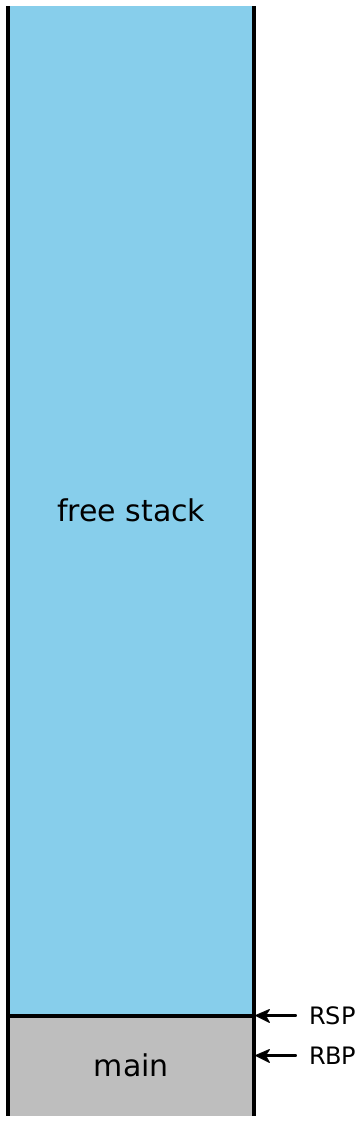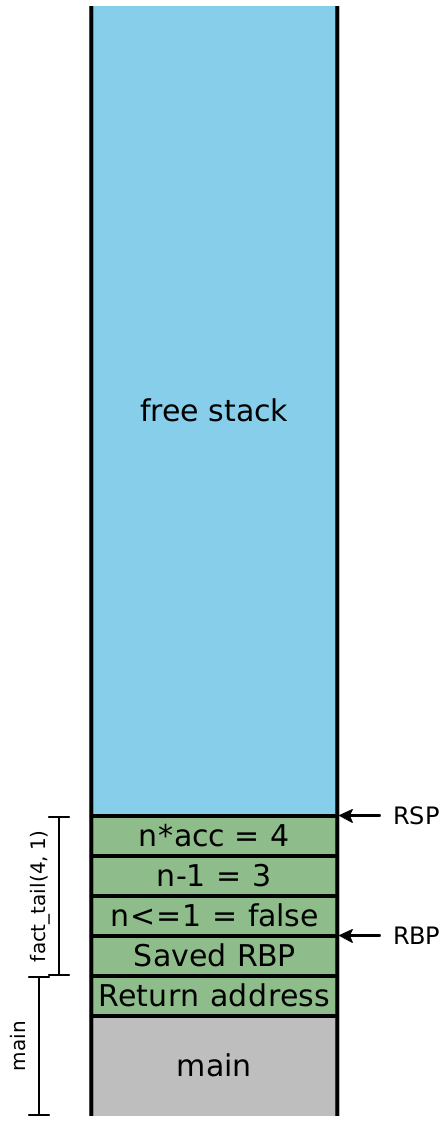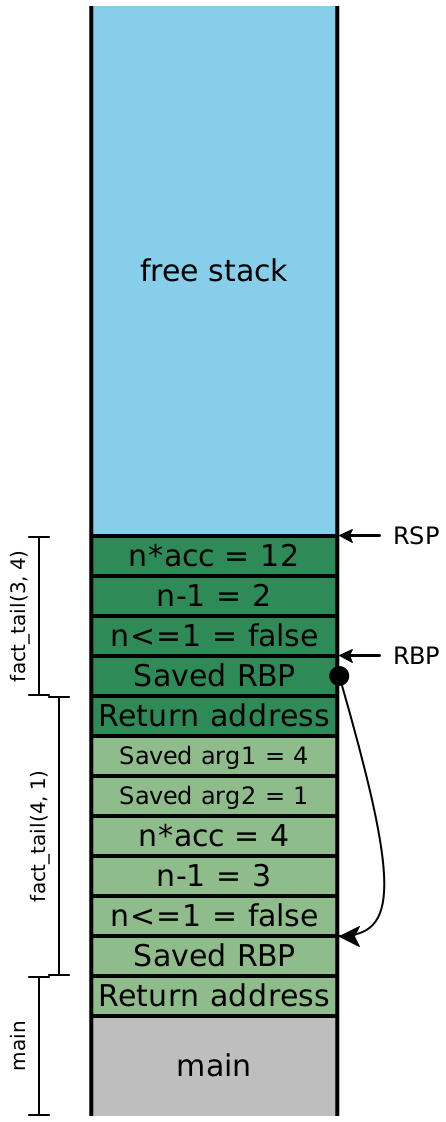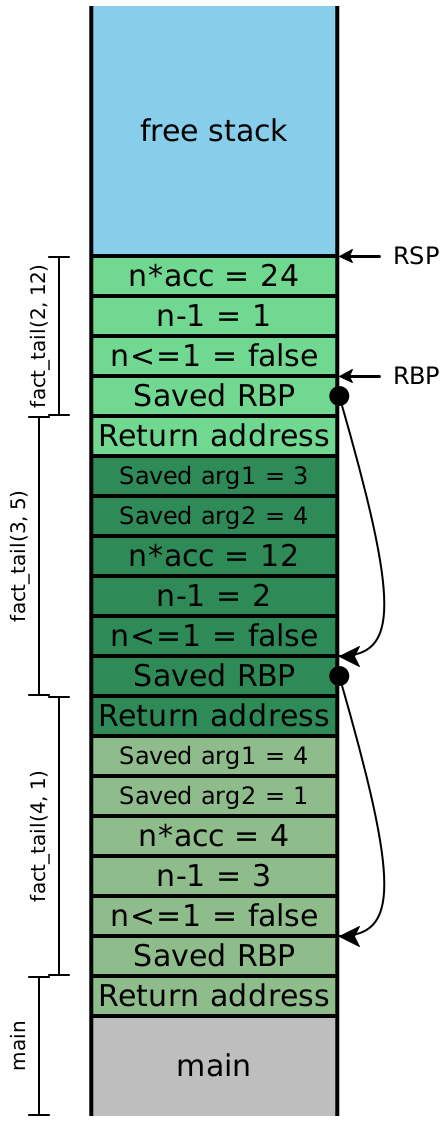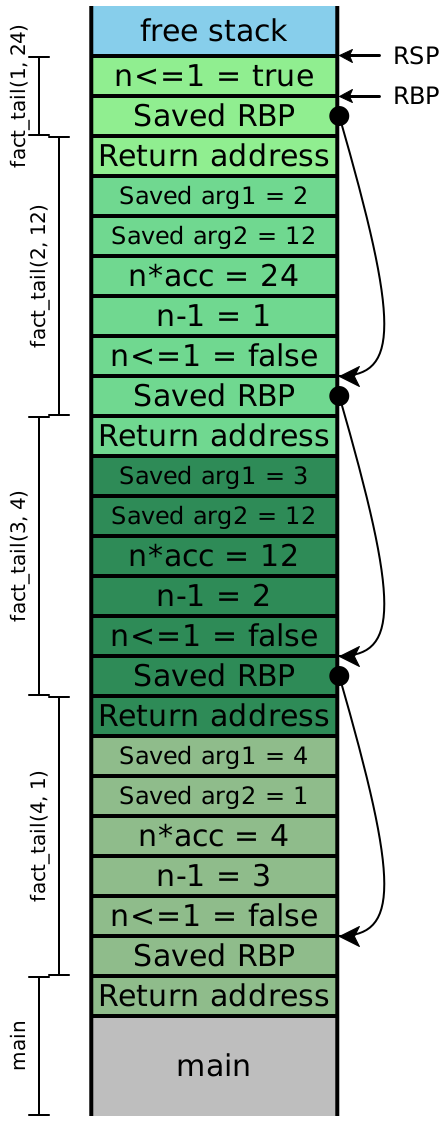Because the recursive calls here are all in tail-position, the next four instructions are all going to be ret instructions, which means the entirety of this stack can effectively be eliminated in one step. In other words, once the olive stack frame makes the call to the dark green frame, we never need to access an olive stack slot again — those carefully-saved values of RDI and RSI aren’t needed. Looking carefully at the stack, we see that the next values for n and acc are precisely the local values computed in the previous stack frame, and moreover, each stack frame has exactly the same shape. If instead of creating a new stack frame, we simply reused the existing one, then we wouldn’t need more than constant stack depth to provide arbitrary call depth!

### 2Strategy (for fewer than 7 arguments)

Rather than saving RDI and RSI before each call by pushing them onto the stack, we can elide that step, and just overwrite their values with the new argument values. Once we’ve done that, we need to re-enter our existing function, but we can’t use call to do it.

Do Now!

Why not?

The meaning of call is to push a return address onto the stack and jump to the destination address. But we already have the necessary return address sitting on the stack! We also have a saved RBP on the stack too, which means that the function prologue we normally execute isn’t really needed here. So instead, we’ll simply jump directly to the next instruction in our code. The compiled assembly for fact_tail would then look roughly like this (ignoring all tag checks, and simplifying the code slightly):

fact_tail:
fact_tail_prologue:
push RBP
mov RBP, RSP
sub RSP, 16           ; reserve stack slots
fact_tail_body:
mov RAX, RDI          ; load n
cmp RAX, 2            ; compare to representation of 1
jg keep_going
mov RSP, RBP          ; and return directly
pop RBP               ; to the original
ret                   ; caller
keep_going:
mov RAX, RDI          ; \
sub RAX, 2            ; | compute n - 1
mov [RBP - 8], RAX    ; /
mov RAX, RDI          ; \
sar RAX, 1            ; |
imul RAX, RSI         ; | compute n * acc
mov [RBP - 16], RAX   ; /
mov RAX, [RBP - 8]    ; \
mov RDI, RAX          ; / OVERWRITE argument n
mov RAX, [RBP - 16]   ; \
mov RSI, RAX          ; / OVERWRITE argument acc
jmp fact_tail_body    ; AND RESTART fact_tail

This code is almost legible enough that we could turn it into C code pretty easily:

int fact_tail(int n, int acc) {
while (true) {
if (n <= 1) { return acc; }
else {
int temp1 = n - 1;
int temp2 = n * acc;
n = temp1;
acc = temp2;
}
}
}

We’ve turned our (tail-)recursive function into a while-loop, and eliminated all the function calls!

### 3Implementation pitfalls

#### 3.1Reusing arguments

Consider the following code:

def max(x, y):
if y >= x: y
else: max(y, x)

This is clearly tail-recursive, so we can apply the same technique above. Since we have no intermediate expressions (again, simplifying the conditional), we don’t even need to move RSP at all; all our values are already on the stack:

max:
max_prologue:
push RBP
mov RBP, RSP
max_body:
mov RAX, RSI    ; load y
cmp RAX, RDI    ; compare to x
jl keep_going
mov RSP, RBP    ; and return directly
pop RBP         ; to the original
ret             ; caller
keep_going:
mov RAX, RSI    ; \
mov RDI, RAX    ; / OVERWRITE argument x
mov RAX, RDI    ; \
mov RSI, RAX    ; / OVERWRITE argument y
jmp max_body    ; AND RESTART max

Do Now!

What went horribly wrong?

Exercise

Try to fix it.

Try tracing through two simple calls to max, to test both branches of the if expression, and carefully step through the generated assembly. If we call max(10, 20), then we fall through the jl instruction, and end up returning RSI, which is y as expected. But suppose we try max(20, 10). then we fall through to keep_going, where we load the current value of RSI and overwrite RDI with it, which effectively copies y into x. Then we load the current value of RDI and copy it into RSI, in an attempt to copy the current value of x into y but at this point, the value of x is gone! So the effect of our tail-call of max(y, x) is to call (10, 10), which then executes the first branch of the conditional and returns 10.

(Note that if we updated our arguments in the other order, such that we overwrote y before we overwrote x, we would have an even more insidious problem: This particular function would compute the correct answer! Our call to max(10, 20) would effectively call max(20, 20) and return 20 purely coincidentally the correct answer. If we changed our program to compute the minimum instead, then this reversed argument-replacement order would once again cause problems.)

The problem is that our new arguments to the call reside in locations that we are about to overwrite, and we’ve managed to create a cycle from the location of y, to the value of the new argument of x, to the location of x to the value of the new argument of y. Our naive strategy of simply moving arguments was too simple. Instead, we can try any of the following strategies in increasing sophistication (or others, in a similar spirit):

• At the beginning of every function, just copy all the arguments into new local variables, and then never use the arguments directly again. This ensures that we can’t have cycles, as above, so our tail calls will always work. On the other hand, we’ll use twice as much stack space as needed.

• Before every tail call, push all the new argument values onto the stack, then pop them (in the opposite order) into their correct locations. This is safe, but every tail call temporarily uses a bit more stack space than is necessary.

• Check whether any of the argument values come from locations that we’re about to overwrite. If so, use the push/pop approach above; if not, use the simpler mov approach.

• Check whether there exists a cycle between new argument values and their locations, as in this max example. For each cycle, break the cycle by pushing one value onto the stack, then mov the remaining arguments as needed, then pop the last argument of the cycle into its place. For any other arguments, just mov them as needed.

The last strategy above is optimal: it never uses more that one extra stack slot at a time, and it uses the minimum number of movs and stack operations. But it’s also clearly the most complicated, and therefore the hardest to test and guarantee correct. The next-to-last strategy strikes a good balance between efficiency and simplicity: the safety condition is easy to check, and both the push/pop-based code and the mov-based code handle all arguments in a uniform manner, making it much easier to test.

#### 3.2Changing arities

The technique above is not limited to self-recursion; it works for tail-calls between functions as well, meaning that mutually recursive functions can also be compiled to essentially a while-loop with a few conditions inside it.

However, the technique above works smoothly only for tail calls to callees whose arities are no greater than their callers’ arities. Suppose function F calls function G, whose arity is $$A_G$$. Suppose G then tail-calls another function H with arity $$A_H > A_G$$. We have two problems:

• First, there isn’t enough room to replace the existing arguments with the intended new ones. We’d need to shift the saved RBP and return address up by a few stack slots, which themselves might be in use (those might well be the new argument values we want to use!), so we’d have to move them as well. This could easily get expensive.

• Second, and more importantly, consider what happens when H finally returns to F (note: G is no longer present; that’s the point of a tail call). F will pop off the $$A_G$$ arguments it pushed onto the stack...but there are actually now $$A_H$$ arguments, and so RSP will wind up in the wrong place! In other words, the calling convention we’ve described so far is incapable of supporting tail-calls to greater-arity functions.

Obviously, these difficulties are not insurmountable, but they do require some clever thought...